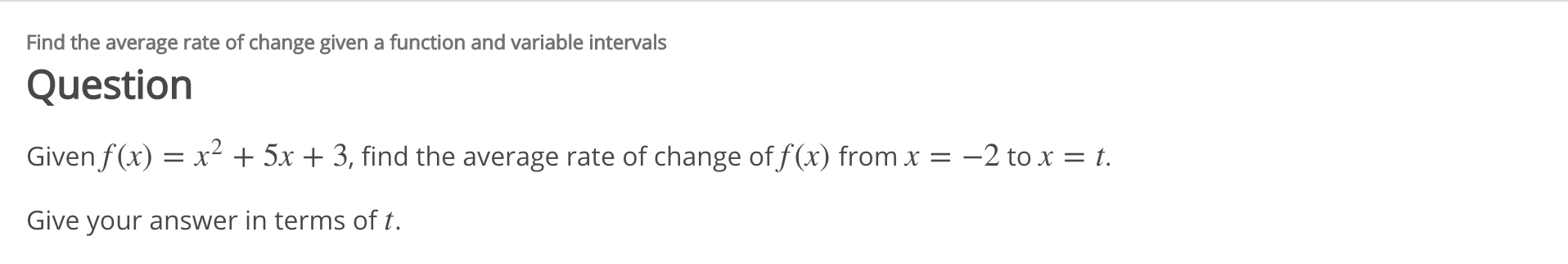# Find the average rate of change given a function and variable intervalsQuestionGiven f(x) = x² + 5x + 3, find the average rate of change of f (x) from x = –2 to x = t.Give your answer in terms of t.

Question
1 viewshelp_outlineImage TranscriptioncloseFind the average rate of change given a function and variable intervals Question Given f(x) = x² + 5x + 3, find the average rate of change of f (x) from x = –2 to x = t. Give your answer in terms of t. fullscreen
check_circle

Step 1

To calculate the average rate of change of a given function from x = -2 to x = t.

Step 2

The average rate of change of a function y = ...

### Want to see the full answer?

See Solution

#### Want to see this answer and more?

Solutions are written by subject experts who are available 24/7. Questions are typically answered within 1 hour.*

See Solution
*Response times may vary by subject and question.
Tagged in

### Derivative